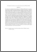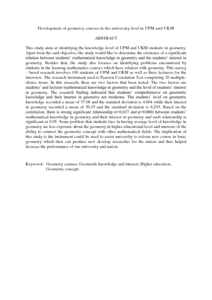Simple Search:

Development of geometry courses in the university level in UPM and UKM

Citation

Abdul Haddy, Fatin and Kilicman, Adem (2013) Development of geometry courses in the university level in UPM and UKM. Menemui Matematik, 35 (1). pp. 56-63. ISSN 2231-7023

Abstract / Synopsis

This study aims at identifying the knowledge level of UPM and UKM students in geometry. Apart from the said objective, the study would like to determine the existence of a significant relation between students’ mathematical knowledge in geometry and the students’ interest in geometry. Besides that, the study also focuses on identifying problems encountered by students in the learning mathematics courses which have relation with geometry. This survey – based research involves 100 students of UPM and UKM as well as three lecturers for the interview. The research instrument used is Pearson Correlation Test comprising 20 multiple-choice items. In this research, there are two factors that been tested. The two factors are students’ and lecturer mathematical knowledge in geometry and the level of students’ interest in geometry. The research finding indicated that students’ comprehensive on geometric knowledge and their interest in geometry are moderate. The students’ level on geometric knowledge recorded a mean of 37.08 and the standard deviation is 4.664 while their interest in geometry recorded a mean of 36.85 and the standard deviation is 6.293. Based on the correlation, there is strong significant relationship (r=0.637 and p=0.000) between students’ mathematical knowledge in geometry and their interest in geometry and such relationship is significant at 0.05. Some problem that students face in having average level of knowledge in geometry are less exposure about the geometry in higher educational level and unaware of the ability to connect the geometric concept with other mathematical fields. The implication of this study is the instrument could be used to assist university to reform new course in basic geometry which then can produce new develop researcher for the nation and thus helped increase the performance of one university and nation.Preview
PDF (Abstract)
Development of geometry courses in the university level in UPM and UKM.pdfView Item